Tuesday, 27 Sep 2022

# What is a point-slope form calculator ?

As you probably already know, there are various ways in which you can represent a straight line in math. So, you can express it in terms of the intercept, the slope, or the independent variable. However, the basic equation of a straight line is y = mx + c. Here y is the dependent variable and x is the independent variable. So, the value of x directly decides the value of the y coordinate. However, neither is x the x-intercept of the straight line nor is y the y-intercept of the same. Therefore, c is the y-intercept of the straight line and m is the value of the slope. Both m and c can be positive or negative. There are various ways to write the equation such that it suits the given data at hand. So, a point-slope form calculator will include the point-slope form of the equation.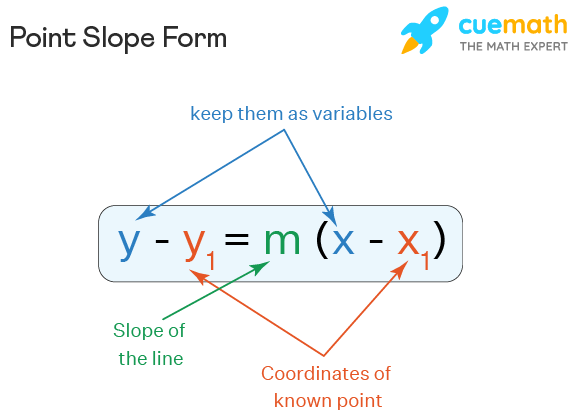The equation of the point-slope form is y- y1 = m (x – x1). So, here y and y1 are 2 points on the y-axis. Similarly, x and x1 are the two points on the x-axis and m is the slope of the line. However, x, y is a random point on the line while x1 and y1 surmise the point given through which the straight line passes. Therefore, you can also use this form to find out the slope when you have two points on the straight line. However, the formula that a point-slope form calculator uses comes from the definition of slope.

So, the slope is the ratio of the difference of the y-coordinates and the difference of the corresponding x-coordinates. So, slope (m) = (y- y1) / (x- x1). Therefore, if we interchange the terms and multiply m with (x – x1), we get (y-y1). This is how a point-slope form calculator functions.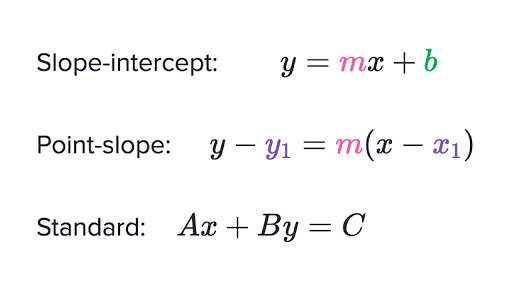## Point-slope form calculator Emath

Calculating the slope of a straight line can often be a little tricky. This is because there are so many options and you never know what kind of data you will have. So, you may have two points or the equation of the straight line to find out the slope. On the other hand, you may have the slope and need to find a point. So, it is only natural that we will be overwhelmed. This is why we should use a point-slope form calculator.Read Also: Improper Integral Calculator

It is lucky that in times such as these, everything is available on the web that makes calculations way easier. So, various online point-slope form calculators can help you out. You just have to enter the data and wait for the results to show up. Moreover, you will also get a full length of steps that are involved in the calculation. So, this is because finding these values is not a one-liner step. Hence, a point-slope form calculator makes sure that you do not just copy a value but understand the process behind the solution. However, different point-slope form calculators are available online. In this case, we will be using the point-slope form calculator of Emath. Click on “Emath” and it will guide you to a link that will land you on the main page of the calculator.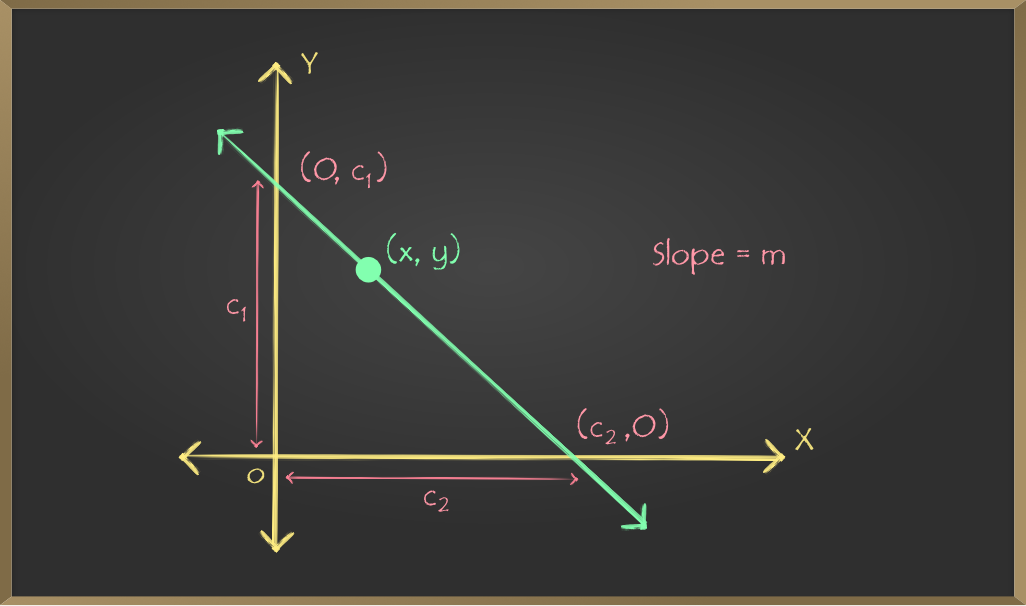## Point-slope form calculator with steps

So, as you can well imagine, there are largely two ways by which you can feed data into a point-slope form calculator. Therefore, you can either enter two points or the equation of a straight line. So, in either case, the point-slope form calculator will give you the value of the slope of the line. Therefore, let us see the steps for both cases.

### Step 1 to use a point-slope form calculator

So, when you open the page, right in the center of the page you find the calculator. Now, you find there are two panels in the point-slope form calculator. In the first panel, two boxes look like search engines. Here you have to feed in the data that you have to the calculator. Therefore, the panel underneath is where you see the solutions.

So, in the first panel, the first box reads “choose type”. There are two in-built types. So, you have to click on either line or two points according to your needs. Let us say, we consider two cases. Therefore, the line is case 1 and two points in case 2.

### Step 2 to use a point-slope form calculator

So, now that you have entered the kind of data that you have, there can be two scenarios. Therefore, in either case, you have to fill up the boxes completely to get the correct answer.

In case I, you have the data that is the equation of a line. So, right under the choose the type box, you will find that there is another box. Therefore, it reads “enter a line”. So, here you have to enter the equation of the line you have. Let us consider an example. So, you enter y = x – 5.

Now, in case II, you have chosen to enter the two points. So, now you have a set of four boxes where you have to enter the values of the two points. Each point gets a set of boxes. So, you write the x-coordinate in the first box and the y-coordinate in the second. Let us say the two points we consider are (3, 4) and (5, 6). So, in the first set of boxes, you enter 3 and 4 individually and then 5 and 6- also individually. So, now you have fed all the information you had to the point-slope form calculator.

However, there is an interesting feature here. When you choose to enter the two points, there is a tab on the panel telling “generate values”. So, if you click on this, the point-slope form calculator automatically generates two points. Moreover, you can get the solution too. So, it is a great option if you are not solving a particular sum as well. Therefore, you can simply use it to understand the concept.

### Step 3 to use a point-slope form calculator

Now, the third step is the same for both cases. So, you have already entered all the information that you had into the calculator. Therefore, at the bottom of this panel, you have a blue tab that reads “calculate”. So, click it to get the final answer as well as the full solution process for either case.

### Step 4 to use a point-slope form calculator

So now, your job is done. Scroll down to the solution panel below. It shows the data that you entered, the entire process, how the calculator has solved it and the final answer as well, separately.

So, in case I, the calculator simply equates with the standard form of a straight line. Therefore, y = x – 5 when represented, y = mx + c, m or the slope is 1. So, the answer is 1.

Now, in case II, the calculator first combines the 4 values into two points. So, they consider P to be (3, 4) and Q to be (5, 6). Therefore, the point-slope form calculator now applies the formula to find the slope. So, slope m = (y2 – y1)/ (x2 – x1). Therefore (y2, x2) and (y1, x1) are (5, 6) and (3, 4) respectively. Hence, the slope is 6-4 / (5-3) = 2/ 2 = 1. So, the final answer is 1.

### Step 5 to use a point-slope form calculator

The point-slope form calculator is also open to comments and suggestions. It may so happen that you wanted to verify an answer but you are quite sure that the calculator is giving a wrong answer. So, in that case, you have the option to give feedback regarding your issues and the calculator will get back to you.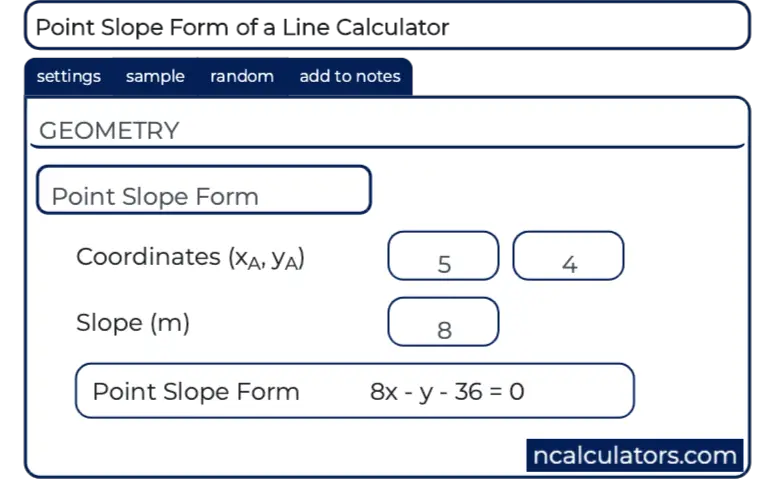### Point-slope form calculator with two points

So, the most common way we use a point-slope calculator is when we have two points through which a straight line passes and we need to find out the slope of the line. So, the point-slope form calculator from Emath allows this. In the direction of what the steps are to operate the calculator, we have already seen how this functions. However, we will take another example. So, this will show us how the operation takes place such that you can have the slope’s value.

Therefore, you can either have two points, or you might want the calculator to generate them for you if you are only interested in knowing the process.

So, you have to enter all the coordinates separately. Then, the calculator groups them into points accordingly. Now, they use the original formula to find the slope of a straight line that needs two points. However, when you are putting the two points, insert the values carefully. This is because the way you enter, the point-slope form calculator calculates as it is. Therefore, if you are not careful enough, there can be an unwanted change in the sign of the line. This is because the direction concerning which you want to find the slope is important.### Point-slope form calculator with fraction

Now, when you are entering the values of the points into the point-slope form calculator, as long as the point exists on the graph, there are no restrictions. So, there are infinite points on a graph even though they cannot properly fit in a single box. Therefore, this means your points can be in fractions and decimals as well. It is not necessary for them to always be integers- either positive or negative. So, let us take an example. Let us say you entered the points ( 3/5, 4/3) and ( 5/2, 6/7).

So, the solution that you will get will consider the two points as P and Q respectively.

Therefore, the points are x1 = 3/5 and y1 = 4/3.

On the other hand, x2 = 5/2 and y2 = 6/7.

Now the slope of a line that passes through 2 points P ( x1, y1) and Q (x2, y2) is m = (y2 – y1)/ (x2- x1)

So, if we put the values properly, what we find is ( 6/7 – 4/3) / (5/2 – 3/5). Therefore, this gives (-10/21) / (19/10). So, this yields -100/ 399. Therefore, this is approximately m = −0.25062656641604. Moreover, the slope, in this case, is negative.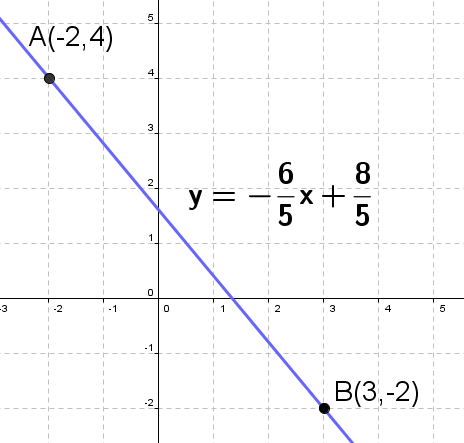### Point-slope form calculator with x-intercept

So, as per definition, the x-intercept is where a straight line crosses the x-axis. Therefore, technically, at this point y must be equal to 0 because the point lies on the x-axis. Hence, you should not be worried if you have to enter the x-intercept to the point-slope calculator for finding out the slope. It is quite easy. So, when you are entering this particular point, you put the value to the x- coordinate. On the other hand, the y-coordinate must be 0. Therefore, let us take an example.

So, let us say one point is (3, 4). The other point that you are entering into the point-slope form calculator is the x-intercept at 5. Therefore, the point is (5, 0). So, from now on, the calculator follows the normal process to find the slope that cuts through the x-intercept and the point (3, 4). Therefore, it considers (3,4) to be P ( x1, y1) and (5, 0) to be Q (x2, y2). So, the slope is m = y2 – y1/ (x2 – x1). This is equal to (4 – 0)/ (3- 5). So, the slope is 4/(-2). Therefore, the final answer is -2.### Point-slope form calculator perpendicular

So, there is also a perpendicular and parallel calculator. Therefore, here, you first have the option to choose whether the line you are finding is perpendicular or parallel to the reference line. After this, you have to enter the equation of the reference line. So, finally, you have to enter the coordinates of the point on the reference line through which the parallel or the perpendicular line passes. This is how it works. However, if you need to find the line equation you can use a line calculator. Similarly, if you need to find the slope of the line, you can go for the point-slope form calculator. It is just one click away.

Moreover, the point-slope form calculator, in this case, might be important. This is because two parallel lines will always have the same slope. Moreover, for perpendicular lines, their slopes are the negative reciprocal of the other one.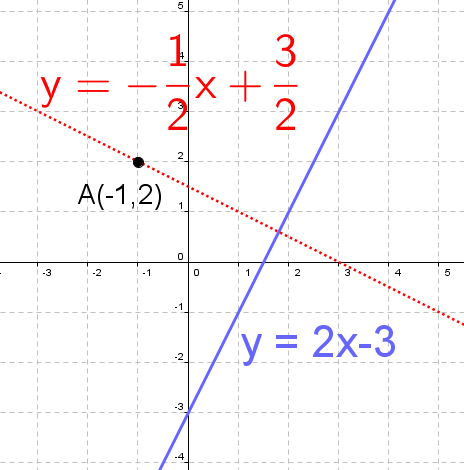### Point-slope form calculator FAQs.

#### What are the rules for point-slope form?

Ans. So, there are no such rules in the point-slope form calculator. However, there are a few requirements. It works following the principle m (x – x1 ) = y – y1. Therefore, you should know any 2 pieces of information. So, you can either have the two points to calculate the slope. Or, on the other hand, you might have a point and the slope to find the coordinates of the other point.

#### Where does point-slope form come from?

Ans. So, this form comes from the basic concept of a slope. Therefore, a slope is the ratio of the difference between 2 y-coordinates with the difference between 2 x-coordinates. So, if you swap the ratio, it adjusts to the equation of a straight line that is y = mx + c. Therefore, we get the point-slope form of the straight line that is m (x – x1 ) = y – y1.#### How do you graph a point-slope equation?

Ans. So, from the point-slope equation, you can easily find the slope of the straight line. Now, plot the point on the graph and follow the slope that you obtained. Therefore, now use the same equation to find 3 or 4 other points. Take arbitrary values of x and find out y from there. Hence, plot all of them. So, you must find your required straight line on the graph.

#### What is the difference between the point-slope form and the slope-intercept form?

Ans. So, the point-slope form calculator considers the points through which the line passes and its slope. On the other hand, the slope-intercept form considers both the x and y-intercepts of the straight line and its slope.

#### What does point-slope form look like?

Ans. So, the point-slope form has a very simple equation that the point-slope form calculator uses. Therefore, it is m (x – x1 ) = y – y1. Here, m is the slope of the line and the rest are the two coordinates (x1, y1) and (x, y).

#### What are the three slope forms?

Ans. So, the three basic slope forms of every linear equation are point-slope form, standard form, and slope-intercept form.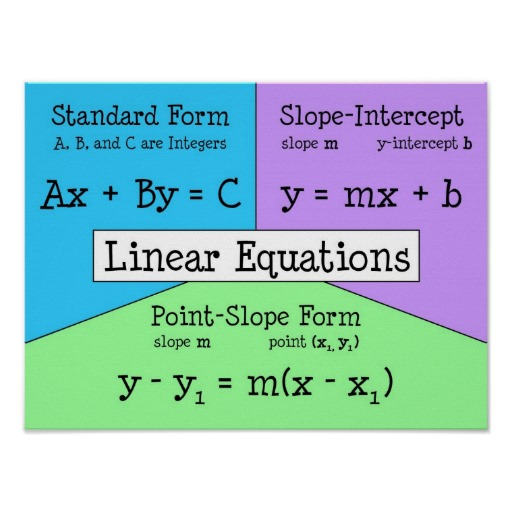#### How do you change point-slope form into standard form?

Ans. To convert the point-slope form into the standard form of a straight line, the extra information that you need is the y-intercept. So, you can easily find it out by putting x = 0 for a value on the y- axis. Now, you already have the slope. Just put the values appropriately on the equation y = mx + c. This is the standard form of the straight line.

#### Is the point-slope form the same as the standard form?

Ans. No, the point-slope form and the standard form of a straight line are not the same. However, they are quite similar. Moreover, the point-slope comes from the standard form of the straight line only.

#### How do you simplify point-slope form?

Ans. So, you can simplify the point-slope form by swapping the equation and finding the slope from it, if you know the coordinates of the two points. If not, then you just match your straight line equation with the standard form of the straight line.

#### When can the slope of a line be 0?

Ans. The slope of a line is 0 when it is horizontal and coincides with the X-axis. So, what this means is the value of the y-coordinate must be 0. Therefore, any line parallel to the x-axis will also have a 0 slope. So, they will have the same y-coordinate which will become 0 when they are subtracted from each other.